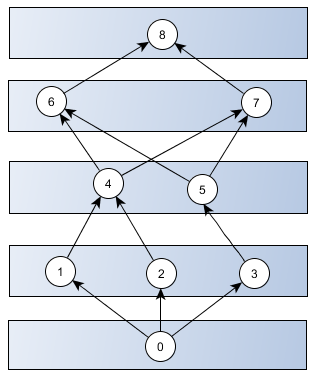## Archive for June, 2012

### Kites on a zebra crossing: an algorithmic challenge

Posted in Algorithms on June 9th, 2012 by kay – Be the first to comment

### Forget Sudoku

A couple of moons ago I stumbled across an algorithmic puzzle which I want to share. It is not too simple s.t. an efficient solution can easily be seen but also not too complicated s.t. it is beyond hope of finding a solution in polynomial time – at least not yet or not for me.

So my effort in describing this problem can also be considered as a crowd sourcing attempt in finding a solution and I call you for contribution. The problem is an idealization of a real algorithmic problem I was dealing with but I stripped off some additional complications in order to improve it as a game play.

Let’s go in medias res quickly.

### The kite and zebra crossing

The basic setup is that of a directed acyclic graph which is layered or stratified as depicted in the diagram below.The "kite on a zebra crossing" diagram

If we enumerate the stripes in ascending order,  a vertex of stripe i can be connected with one or more vertices in stripe i+1. There is a single vertex in the bottom stripe and the top stripe. Each path from the bottom vertex to the top vertex has the same length and each move upwards is as good as any other.

In order to create a challenge we complicate the situation. For a given positive number K we assign a possibly empty list of numbers with elements in {-K, -K+1, … ,K-1, K} – {0} with each vertex V in the kite. So for example we set K = 2 and make the assignments

```V.numlist = []
V.numlist = [-1, -2]
V.numlist = [-1, -1]
V.numlist = [-1]
V.numlist = 
V.numlist = 
V.numlist = 
V.numlist = [-2]
V.numlist = []```
Number lists can assigned to paths of the kite as well. Let Pt(i, j) an arbitrary path from V[i] to V[j]. The number list of Pt(i,j) is the concatenation of the number list of its vertices. Examples for such paths and their associated number lists are
```Pt(V, V, V, V, V).numlist = [-1, -1, 2, -2]
Pt(V, V, V, V, V).numlist = [-1, -2, 2, 1]```
On number lists we define a reduction rule which states:

Remove adjacent numbers x, y in a number list if x+y = 0

In the number list [-1, -2, 2, 1] we can first remove -2 and 2 and get [-1, 1] which can be further reduced to []. The number list [-1, -1, 2, -2] can be reduced to [-1, -1] but not any further.

We write

L1 => L2

if there is a sequence of reductions of the number list L1 yielding L2 and say that a complete path Pt(V, V[N]) connecting the bottom with the top of the kite is valid iff  Pt(V, V[N]).numlist => []. The number list of a valid path can be reduced to the empty list.

### Now the challenge

Assume that K is fixed and let the number N>=2 of kite vertices be variable. So we consider the set of all kites which are constrained by N vertices and this for arbitrary numbers N>=2. Is there an algorithm which can determine a valid path ( or detect its absence ) which is polynomial in N?